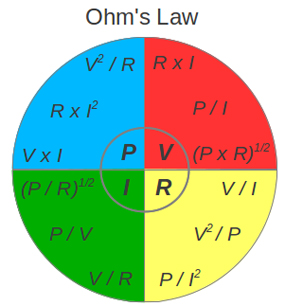# Design & Engineering support for modern electro-mechanical systems.

Hydrotech isn't just a distributor of quality industrial parts, We staff a full engineering department with experienced experts ready to design, assemble and integrate full system solutions.

# Electrical Technical Data### Electrical Units

#### Common electrical units used in formulas and equations are:

• Volt - unit of electrical potential or motive force - potential is required to send one ampere of current through one ohm of resistance
• Ohm - unit of resistance - one ohm is the resistance offered to the passage of one ampere when impelled by one volt
• Ampere - units of current - one ampere is the current which one volt can send through a resistance of one ohm
• Watt  - unit of electrical energy or power - one watt is the product of one ampere and one volt - one ampere of current flowing under the force of one volt gives one watt of energy
• Volt Ampere  - product of volts and amperes as shown by a voltmeter and ammeter - in direct current systems the volt ampere is the same as watts or the energy delivered - in alternating current systems - the volts and amperes may or may not be 100% synchronous - when synchronous the volt amperes equals the watts on a wattmeter - when not synchronous volt amperes exceed watts - reactive power
• Kilovolt Ampere  - one kilovolt ampere - KVA - is equal to 1,000 volt amperes
• Power Factor - ratio of watts to volt amperes

## Electrical Formulas

### Electric Power Formulas

P = V I         (1a)

P = R I2         (1b)

P = V2/ R         (1c)

where

P = power (Watts)

V = voltage (Volts)

I = current (Amperes)

R = resistance (Ohms)

### Electrical Potential Formulas - Ohms Law

Ohms law can be expressed as:

V = R I         (4a)

V = P / I         (4b)

V = (P R)1/2         (4c)

### Example - Ohm's law

A 12 volt battery supplies power to a resistance of 18 ohms.

I = (12 Volts) / (18 ohms)

= 0.67 Ampere

### Electric Current Formulas

I = V / R         (2a)

I = P / V         (2b)

I = (P / R)1/2         (2c)

### Electric Resistance Formulas

R = V / I         (3a)

R = V2/ P         (3b)

R = P / I2         (3c)

## Electrical Motor Formulas

### Electrical Motor Efficiency

μ = 746 Php / Pinput_w         (5)

where

μ = efficiency

Php = output horsepower (hp)

Pinput_w = input electrical power (Watts)

or alternatively

μ = 746 Php / (1.732 V I PF)         (5b)

### Electrical Motor - Power

P3-phase = (V I PF 1.732) / 1,000         (6)

where

P3-phase = electrical power 3-phase motor (kW)

PF = power factor electrical motor

### Electrical Motor - Amps

I3-phase = (746 Php) / (1.732 V μ PF)         (7)

where

I3-phase = electrical current 3-phase motor (Amps)

PF = power factor electrical motor

OUR PURPOSE

## Industry in Motion

American industry has never enjoyed more opportunity than it does today – or faced more challenges. Hydrotech is here to help you compete, evolve, lead and win with superior solutions in fluid power, automation, service & repair and connected technologies.